# Low-Noise Amplifier Stability Concept to Practical Considerations, Part 3

### Abstract

Part 3 completes the series by presenting application examples. The first shows how to match an LNA in the maximum available gain condition. The second deals with an LNA matched in the constant desired gain condition. The third exercise stresses the importance of matching a potentially unstable LNA in its stable area.

In Part 1, we started our discussion with a brief background on transmission lines and a reminder about RF power gain definitions. Next, in Part 2 we jumped into the RF aspect of low-noise amplifiers by examining stability (tendency for oscillation), impedance matching, and general amplifier design, using scattering parameters (S-parameters) as design tools. Part 3 completes the series by presenting application examples. The first shows how to match an LNA in the maximum available gain condition. The second deals with an LNA matched for constant gain at a desired level. The third exercise stresses the importance of matching a potentially unstable LNA in its stable area.

### Applications

To support the theory of LNA matching with practical examples, we turn first to the MAX2720/MAX2721 PA drivers (Figure 1). These are low-cost, high-performance, direct I/Q modulators designed for use in WCDMA and wireless-local-loop (WLL) systems. When compared with devices featuring dual-conversion architectures, their direct up-conversion architecture reduces the system cost, component count, and board space.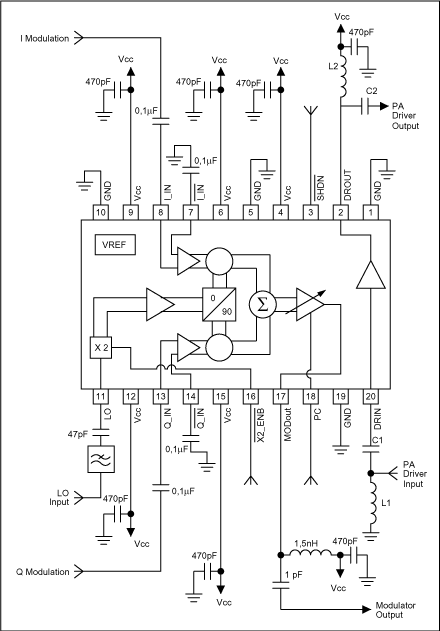Figure 1. Typical operating circuit for the MAX2721 PA driver.

The MAX2720 and MAX2721 include an I/Q modulator, a variable gain amplifier (VGA), and a power amplifier (PA) driver. The quadrature modulator accepts differential baseband I/Q signals and directly modulates an RF carrier in the 1.7GHz to 2.1GHz range (MAX2720) or the 2.1GHz to 2.5GHz range (MAX2721).The first application example features a MAX2721 PA driver used in the maximum-available-gain condition at 2.3GHz. The PA driver must operate between 50Ω terminations. As explained in chapter 10, this requirement means that the MAX2721's input and output matching networks must meet a simultaneous conjugate-match condition. The MAX2721 has the following S-parameters (magnitude/angle) at 2.3GHz:

S11 = 0.456/135.8°
S21 = 3.176/80.4°
S12 = 0.11/75.9°
S22 = 0.051/-133.9°

The PA driver is usable in the maximum-available-gain mode only if unconditionally stable at the 2.3GHz operating frequency. Before beginning any design calculation, first use Eqs.7-8 and 7-9 to check whether the stability coefficient K is larger than one.The intermediate value is: Magnitude |ΔS| = |S11S22 - S21S12| = 0.370. Angle = -22.146°

Use the intermediate value to calculate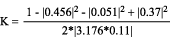= 1.326.

Because K is larger than 1 and |ΔS| less than 1, the PA driver is unconditionally stable, and we may proceed. The maximum available gain is given by Eq. 10-8:An external PA driver would be needed if the design specification called for a minimum gain larger than 11.185dB. We will consider 11.185dB adequate for our purpose. The next step is to find the load reflection coefficient needed for a conjugate match.

First, the two intermediate quantities C2 and B2 must be found. From Eq. 9-7,

C2 = (0.051/-133.9°) - (0.456/-135.8°)(0.370/–22.146°) = 0.124/12.416°.

Also from Eq. 9-7,

B2 = 1 + (0.051)² - (0.456)² - (0.370)² = 0.657.

Thus, the magnitude of the load-reflection coefficient can be found using Eq. 9-12. Because B2 > 0, the minus sign can be used before the radical in the reflection coefficient:The angle of the load reflection coefficient is equal to the negative of the angle of C2:

ΓL = 0.196/-12.416°

The next step is to find the source-reflection coefficient needed for a conjugate match. First, the two intermediate quantities C1 and B1 must be found. From Eq. 9-4,

C1 = (0.456/135.8°) - (0.051/133.9°)(0.370/–22.146°) = 0.439/136.805.

Also from Eq. 9-4,

B1 = 1 + (0.456)² + (0.051)² - (0.370)² = 1.068.

Therefore, the magnitude of the source-reflection coefficient can now be found using Eq. 9-13. Because B1 > 0, the minus sign can be used before the radical in the reflection coefficient:

The angle of the source reflection coefficient equals the negative of angle C1:

Γs = 0.523/-136.805°

Once the desired ΓS and ΓL are known, all that remains is to surround the PA driver with components that provide source and load impedances that "look like" ΓS and ΓL. Design of the input matching network is shown on the Smith Chart in Figure 2. The object of the design is to force the 50Ω source to present a reflection coefficient of 0.523/-136.805°.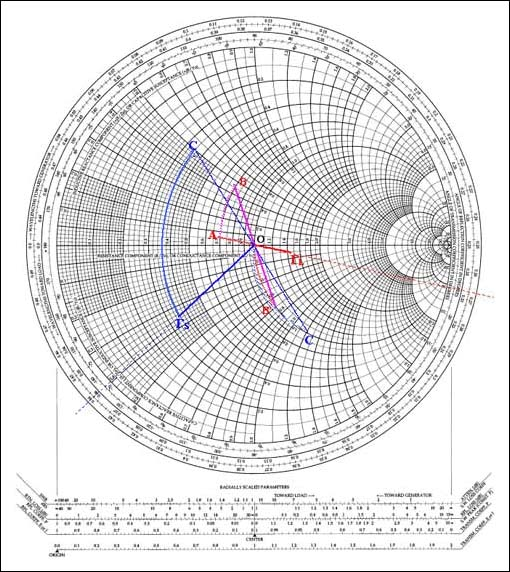Figure 2. Smith Chart illustrating the input and output impedance matching necessary for a MAX2721 PA driver to operate with maximum available gain.

With ΓS plotted in blue as shown, the desired, normalized, corresponding impedance is read directly from the chart as ZS = 0.36 – J0.36Ω. Remember, the impedance is normalized because the chart has been normalized to 50Ω. The actual impedance represented by ΓS equals 50(0.36 – J0.36)Ω = 18 – J18Ω. To force the 50Ω source to appear as an 18 – J18Ω impedance to the PA driver, we merely add a shunt and a series reactive component as shown on the chart in Figure 2. Proceeding from the source, we have a shunt inductance (L1), so we have to start in the admittance chart.

Start from zero on the Smith Chart, which is the normalized 1(1/50Ω) source admittance, and turn counter-clockwise, because inductance in shunt produces the negative admittance of a quantity yet to be determined. Seen from the PA driver input on the plot, the end point must be situated on a circle of constant r = 1, such that the C1 serial capacitor (counter-clockwise rotation in the impedance chart) will end at the point ΓS.

Arc OC gives the value of the shunt inductance L1.
Arc C'ΓS gives the value of the serial capacitor C1.

The measured value of the arc OC is 1.2 units, so Z = 50/1.2 = 41.66Ω. Thus, L1 = 41.66/ω = 41.66/2π f = 41.66/2π (2.3 × 109) = 2.88nH, rounded to 3nH. The measured value of the arc C'ΓS is 0.86 units, so Z = 50 × 0.86 = 43Ω. Thus, C1 = 1/(43ω) = 1/(43 × 2π f) = 1/(43 × 2π × 2.3 × 109) = 1.609pF, rounded to 1.6pF. This completes the input-matching network.

The load-reflection coefficient is plotted in red on the Smith Chart (Figure 2). As read from the chart, it represents a desired load impedance of ZL = 50(01.47 -J0.13)Ω = 73.5 – J6.5Ω. The matching network is designed as follows, proceeding from the load:

On the Smith Chart start from zero, which is the normalized 1(50Ω) load impedance. Turn counter-clockwise, because the serial capacitor C2 implies the negative reactance of a quantity yet to be determined. The end point must be situated on the circle of constant r = 1, so the L2 shunt inductance (counter-clockwise rotation in the admittance chart) ends at point A on the plot.

Arc OB' gives the value of the serial capacitor C2.
Arc AB gives the value of the shunt inductance L2.

The measured value of arc OB' is 0.7 units, so Z = 50 × 0.7 = 35Ω. Thus, C2 = 1/(35ω) = 1/(35 × 2π f) = 1/(35 × 2π × 2.3 × 109) = 1.977pF, rounded to 2pF. The measured value of arc AB is 0.4 units, so Z = 50/0.4 = 125Ω. Thus, L2 = 125/ω = 125/2π f = 125/(2π × 2.3 × 109) = 8.649nH, rounded to 8.2nH.

On the MAX2721 block diagram, note that the 470pF capacitor between ground and the junction of VCC and L2 is considered a short circuit at 2.3GHz. This completes the output-matching network.

### Constant-Gain Application

A second application employs the MAX2721 PA driver in an 8dB constant-desired-gain condition at 2.3GHz. The PA driver must operate between 50Ω terminations. As explained in chapter 11, we match the PA driver input and output impedances by defining a constant-gain circle on the Smith Chart. The PA driver is unconditionally stable with K = 1.326.

Using Eq. 11-10 and proceeding "by the numbers," we locate the center of the circle at a point:Center is therefore located at 0.085/-12.416°.

This point can now be plotted on the Smith Chart. The radius of the 8dB-gain circle is calculated as:The Smith Chart construction is shown in Figure 3. If the input impedance of the PA driver is conjugately matched, note that any load impedance located along the circumference of this circle produces an amplifier gain of 8dB. As noted in the problem statement, the actual load impedance we have to work with is 50Ω. Its normalized value is shown as the Smith Chart origin (point O) in Figure 3. The PA driver's output network must transform the actual load impedance into a value that falls on the constant gain circle.Figure 3. Smith Chart illustrating the input and output impedance matching necessary for a MAX2721 PA driver to operate with a specified gain (8dB in this case).

Several circuit configurations can accomplish this task. For convenience, we choose the configuration ΓL = 0.68/+118° for the load reflection coefficient (shown in red on the Smith Chart). Proceeding from the load,

Arc A'B gives the value of shunt inductance L1 (counter-clockwise in the admittance chart).
Arc OA gives the value of series capacitor C1 (counter-clockwise in the impedance chart).

The measured value of arc A'B is 1.9 units, so Z = 50/1.9 = 26.31Ω. Thus, L2 = 26.31/ω = 26.31/(2π f) = 26.31/(2π × 2.3 × 109) = 1.82nH, rounded to 1,8nH. The measured value of arc OA is 0.8 units, so Z = 50 × 0.8 = 40Ω. Thus, C2 = 1/(40 × ω) = 1/(40 × 2π f) = 1/(40 × 2π × 2.3 × 109) = 1.8pF, rounded to 1.8pF.

For a conjugate match at the input to the PA driver, with ΓL = 0.68/+118°, the desired source reflection coefficient must be as shown in Eq. 9-1: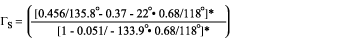The source reflection coefficient Γs is therefore 0.319/-167°.

This point is plotted as Γs in Figure 3. As given in the problem statement, the actual source impedance is 50Ω. Its normalized value is shown as the Smith Chart origin (point O). Thus, the input network must transform the actual impedance at point O to the desired impedance at point Γs. For practice, this was done with a two-element design as shown.

Arc ΓsC' gives the value of series capacitor C1 (counter-clockwise in the impedance chart).
Arc OC gives the value of shunt inductance L1 (counter-clockwise in the admittance chart).

The measured value of arc ΓsC' is 0.42 units, so Z = 50 × 0.42 = 21Ω. Thus, C2 = 1/(21 × ω) = 1/(21 × 2π f) = 1/(21 × 2π × 2.3 × 109) = 3.29pF, rounded to 3.3pF. The measured value of arc OC is 0.93 units, so Z = 50/0.93 = 53.8Ω. Thus, L2 = 53.8/ω = 53.8/(2π f) = 53.8/(2π × 2.3 × 109) = 3.72nH, rounded to 3.8nH.

### Match for Stability

In a third application, the MAX2320 PCS-Band LNA (Figure 4) operates at 13.5dB gain as described in the MAX2320 data sheet under high-gain, high-linearity mode of operation. The MAX2320 (a high-performance, silicon-germanium (SiGe) receiver front-end) is one of a 6-member family of ICs dedicated to dual-band, triple-mode CDMA/TDMA/GSM handset applications. These devices set a new standard for low noise and high linearity at low supply current. They integrate a variety of features, including an LO frequency doubler and divider, gain settings for a dual low-noise amplifier (LNA), and a low-current paging mode that extends the handset standby time.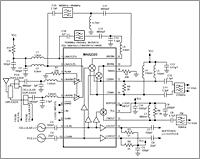For Larger Image

Figure 4. Typical operating circuit for the MAX2320 PCS-band LNA (receiver front end).

As a 50Ω termination, the LNA output is loaded by a Murata bandpass filter of 1960MHz ± 30MHz (LFSN30N15C1960). For matching the LNA input and output, we define on the Smith Chart a circle of constant gain (13.5dB) as explained in chapter 11. The MAX2320 has the following S-parameters (magnitude/angle) at 1.95GHz:

S11 = 0.43/-115°
S21 = 3.82/84°
S12 = 0.09/75°
S22 = 0.673/-57°

We locate the center of the circle at the point:The center is therefore located at 0.633/+63°.

On the Smith Chart (Figure 5), the radius of this 13.5dB-gain circle is calculated as: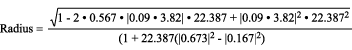A calculation of the stability factor K for the MAX2320 PCS-Band LNA indicates a potential instability at K = 0.567 and ΔS = 0.167. To prevent oscillation, we must therefore exercise extreme caution in choosing source and load impedances for the device. To find stable operating regions on the Smith Chart (Figure 5), we must plot the input and output stability circles. Because |ΔS| is less than |S11| or |S22|, the areas of stability are outside the input and output stability circles.Figure 5. The output of this MAX2320 PCS LNA is matched for a desired gain of 13.5dB.

The only load impedance that we may not select for the LNA is located inside the output stability circle. Any other load impedance located on the 13.5dB gain circle will provide the needed gain, provided the device input is conjugately matched, and provided the input reflection coefficient ΓS required for a conjugate match falls outside the input stability circle.

We choose load reflection coefficient ΓL = 0.6/18° as a convenient value on the 13.5dB gain circle.

Arc ΓLA gives the value of the shunt inductance L2 (counter-clockwise in the admittance chart).
Arc B'O gives the value of the series capacitor C2 (counter-clockwise in the impedance chart).

The measured value of arc ΓLA is 0.56 units, so Z = 50/0.56 = 89.29Ω. Thus, L2 = 89.29/ω = 89.29/(2π f) = 89.29/ (2π × 1.96 × 109) = 7.25nH, rounded to 7.2nH. The measured value of arc B'O is 1.7 units, so Z = 50 × 1.7 = 85Ω. Thus, C2 = 1/(85 × ω) = 1/(85 × 2π f) = 1/(85 × 2π /appnotes/1852× 1.96 × 109) = 0.955pF, rounded to 1pF.

Using Eq. 9-1, calculate the source reflection coefficient ΓS needed for a conjugate match, and plot that point on the Smith Chart (Figure 6):Source Reflection Coefficient: ΓS = 0.521/+147.74°. Note that ΓS falls in the stable region of the input stability circle (Figure 6), and therefore represents a stable termination for the LNA.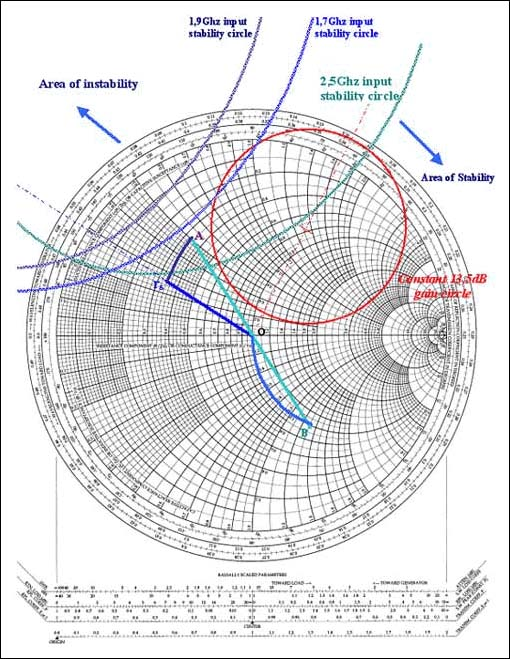Figure 6. The input of this MAX2320 PCS LNA is matched for a desired gain of 13.5dB.

The 6800pF high-value capacitor between the duplexer and inductance L1 is not to be considered in the input-matching calculation, because it assumes only a DC-blocking function for the LNA input. Loaded with a 50Ω antenna, the PCS duplexer shows a 50Ω impedance that must be matched to the LNA input.

Arc ΓSA gives the value of series inductance L1 (clockwise in the impedance chart).
Arc BO gives the value of shunt capacitor C1 (clockwise in the admittance chart).

The measured value of arc ΓSA is 0.22 units, so Z = 50 × 0.22 = 11Ω. Thus, L1 = 11/ω = 11/(2π f) = 11/(2π × 1.96 × 109) = 0.893nH, rounded to 1nH. The measured value of arc BO is 1.3 units, so 1/Y = Z = 50/1.3 = 38.46Ω. Thus, C1 = 1/(38.46 × ω) = 1/(38.46 × 2π f) = 1/(38.46 × 2π × 1.96 × 109) = 2.11pF, rounded to 2.2pF.

Reference
Bowick, Chris. RF Circuit Designs. Howard W. Sams & Co. Inc., 4300 West 62nd Street, Indianapolis, Indiana (a publishing subsidiary of ITT).